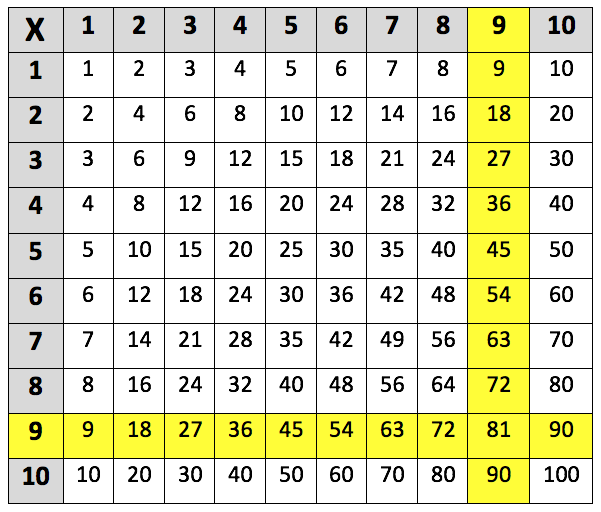Learning the 9 times table. The 9 times table up to 9 x 10 is highlighted in the multiplication grid below:This is the 9 times table up to 9 x 12:
9 x 1 = 9
9 x 2 = 18
9 x 3 = 27
9 x 4 = 36
9 x 5 = 45
9 x 6 = 54
9 x 7 = 63
9 x 8 = 72
9 x 9 = 81
9 x 10 = 90
9 x 11 = 99
9 x 12 = 108

## When are children taught the 9 times table?

During Year 4, children will learn the 6, 7, 9, 11 and 12 times tables. By the end of Year 4, children should have been taught all the times tables up to the 12 times table. Teachers will start giving children harder multiplications to complete, these will include multiplying three-digit numbers by a one-digit number (such as 150 x 3 = 450).

## Are there any tricks to make the 9 times table easier to learn?

One way of remembering whether a number is in the 9 times table is by knowing that the digits of multiples of 9 (up to 90) always add up to equal 9. For example:

27 (9 x 3)        2 + 7 = 9

63 (9 x 7)        6 + 3 = 9

Multiples of 9 which are greater than 90 will still add up to equal a multiple of 9. For example:

99 (9 x 11)       9 + 9 = 18

117 (9 x 13)      1 + 1 + 7 = 9

Recognising these patterns will enable children to check their own answers to questions which ask them to multiply a number 9. It also means if they were given a number, children would be able to easily work out whether it is a multiple of 9.

## How to help children with the 9 times table?

Sometimes it can help children to calculate multiples of 9 by multiplying by 10 and then subtracting the number which they are multiplying by. For example:

What is 6 x 9?

6 x 10 = 60

60 - 6 = 54

Therefore, 6 x 9 = 54.

This breaking down method is useful because children are often more confident in their 10 times tables.

One clever way of practising the 9 times table is by learning how to answer 9 times table questions (up to 9 x 10), by using the ‘hand method’.

This method involves holding up all 10 fingers and then bending down the finger which you are multiplying 9 by. For example:

To work out 4 x 9, bend down the 4th finger of your left hand, there are 3 fingers to the left of this and 6 fingers to the right. Then you simply place these numbers together to get 36. The answer to 4 x 9 = 36.

## How does Learning Street help children with the 9 times table?

Repetition is key to learning your 9 times table, and in the Learning Street 8 year old courses, there is a constant focus on always practising times tables as this is the best way to improve. This is through times tables tests (to improve accuracy), tables races (to improve time), mental mathematics, maths problem solving and other fun activities such as mystery pictures.

Without a good knowledge of times tables, it can lead to problems in SATs, GCSEs and A Levels.

## Our Courses

Click through to review the courses we have available

##### Get Started

Learning Street structured courses make home study easier and more successful. There's no need to wonder which books to buy or what to do next. Everything is planned for you.

• Proven to deliver excellent results
• Used by families, private tutors and schools
• Fully planned and structured